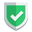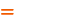我们相信：世界是美好的，你是我也是。平行空间的世界里面，不同版本的生活也在继续...

## 获得每列数据

``````import pandas as pd
import numpy as np

# data_url = "iris.csv"
column_names = ["蕊长", "蕊宽", "瓣长", "瓣宽", "种类"]

column_0 = np.array(df_iris["蕊长"])
column_1 = np.array(df_iris["蕊宽"])
column_2 = np.array(df_iris["瓣长"])
column_3 = np.array(df_iris["瓣宽"])
column_4 = np.array(df_iris["种类"])``````

## 画散点图

``````data_x = column_0
data_y = column_1
data_z = column_4``````

`data_z`就是鸢尾花分类的`ndarray`一维数组，它里面一共有`0/1/2`三种取值，分别对应于`cmap`中的`blue/red/green`颜色设置，按照顺序一一对应。

``````import matplotlib.pyplot as plt
fig = plt.figure("iris data", figsize=(15, 15))
plt.title("Sepal Length * Sepal Width Graph")
plt.suptitle("the graph suptitle\nblue:setosa,red:versicolor,green:virginica")
plt.xlabel("Sepal Length")
plt.ylabel("Sepal Width")
# plt.scatter(data_x, data_y)
q1 = plt.scatter(data_x, data_y, c=data_z, cmap="brg")
plt.show()``````

• 画布尺寸参数`figsize`的单位是英寸。
• `scatter()``c``cmap`，控制了散点的颜色。`brg`的意思就是`blue/red/green`。不传这两个参数的话，就统一显示默认的蓝色圆点。

## 结束语如果本文对您有帮助，或者节约了您的时间，欢迎打赏瓶饮料，建立下友谊关系。本博客不欢迎：各种镜像采集行为。请尊重原创文章内容，转载请保留作者链接。【福利】 腾讯云最新爆款活动！1核2G云服务器首年50元！【源码】本文代码片段及相关软件，请点此获取更多信息【绝密】秘籍文章入口，仅传授于有缘之人python    plot